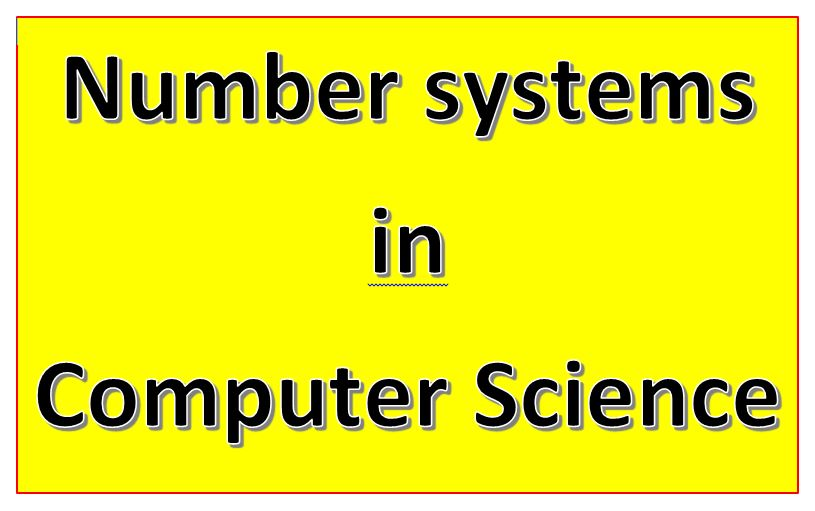In this article, you will learn about the Number System Computer Science Class 11 and their conversion. Number System in Computer Science is very important for representing data in computer systems.

Topics Covered

## The number system Computer Science Class 11

The number system is very useful to represent data with specific symbols and digits. Data representation can be done through numbers. So we can say, it is a way of using numbers with relevant symbols to work upon data. There are three main properties of the number system.

1. The Base: The total number of digits used in the number system Ex. 10 is the base for the decimal number system It is also known as radix.
2. Numbers: The numbers used Ex 0 to 9
3. The Position: Position of each number from right to left also known as LSB (Least significant Bit) and MSB(Most Significant Bit)

## Type of Number System Computer Science Class 11

There are four types of number systems computer science class 11.

## Conversion of Number System Computer Science Class 11

You can convert a number from one system to another number system. There are two methods used for the conversion of numbers.

### Decimal to others

1. Divide the decimal number with the base
2. Note down remainders in each step
3. Write down reminders in reverse order

Ex. Convert (98)10 = (?)2

### Others to decimal

1. Write the position of each number from right to left, start with 0
2. Multiply and the number with raised power of the base value

Ex. Convert (100110)2 = (?)10

### Octal to Decimal

(2577)8=(?)10

= (2 × 83) + (5 × 82) + (7 × 81) + (7 × 80)

= (2 × 512) + (5 × 64) + ( 7 × 8) + (7 × 1)

= 1024 + 320 + 56 + 7

= 1407

(2577)8=(1407)10

(16119)10 = (?) 16

Ans. : (16119)10 = (3EF7) 16

### Fractional part

The fractional part conversion is quite simple to convert. Follow this method to convert:

### Decimal to Binary

1. Multiply the decimal number with the base
2. Note down the integer part and continue step 1 with the fractional part
3. Continue the above steps until the fractional part will be 0 or repeat 5 to 6 steps
4. Write down the number from top to bottom

### Binary to Decimal

Now you convert octal to decimal, hexadecimal to octal and vice versa…

There are some shortcut methods also used to convert octal to binary and vice versa.

You can refer to this tutorial also for the same purpose.

Watch this 1 shot video for more understanding:

As in a Binary system, only 0s and 1s are used to represent the number, addition rules are as follows:

1. 0 + 0 = 0
2. 0 + 1 = 1
3. 1 + 0 = 1

1 + 1 = 10 (0 with carry 1)

Example:

1. 10101 + 10001

2. 11101 + 10101 + 10001

In the above examples, you have seen the common and basic rules for addition. In the examples, C refers to carry on top. In the first example, 1 + 1 = 10, So 0 with carrying 1 is processed and carried forward as usual. In second example, first digit calculation 1 + 1 + 1 i.e. 1 + 1 = 10 then 10 + 1 = 11.  similarly, next digits computations are done. In last position 1 + 1 + 1 + 1 = 1 + 1 = 10 + 1 = 11 + 1 = 100.

Thank you for reading the post. If you have any doubt or queries do not hesitate about it. Post your doubt or query as a comment. We will respond to your comment as soon as possible.

Thank you for reading, enjoy learning!!

## FAQs Number System Computer Science Class 11

### What is number system in computer science class 11?

The number system is one of the topics of the CBSE Computer Science Class 11 Curriculum. There is one chapter on this topic in Computer Science Class 11. Students will learn about the number system and its conversion.

### What is number system in computer system?

The number system is used to represent data in the computer system. Everything we are processing in a computer system gets converted into numbers in the computer system.

### What are some number systems used by computers class 11?

According to the syllabus of computer science class 11 you will learn four number systems:
1. Binary
2. Decimal
3. Octal

### What is decimal number system class 11?

The decimal number system class 11 refers to the number system which we are using for calculation and presenting numbers wherever applicable. Its using 10 digits ranging from 0 to 9, So it has base 10.

##### 3 thoughts on “Comprehensive notes Number System Computer Science Class 11”
1.Adarshya Annanya says:

Thank you so much sir…

1.tutorialaicsip says:

Welcome Anannya

2.Neha says:

Nice